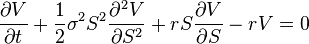# Open Source

Below are several open source programs I have created for research and to showcase my work.

## Black-Scholes CalculatorFind the numerical solution to the famous Black-Scholes Equation using the implicit Crank Nicolson Finite Difference Method.

## Finite Difference Calculator

Calculate numerical solutions to Parabolic, Hyperbolic and Elliptic Partial Differential Equations such as the Wave Equation, Maxwell’s Equations or Black-Scholes Equation using the Finite Difference Method.